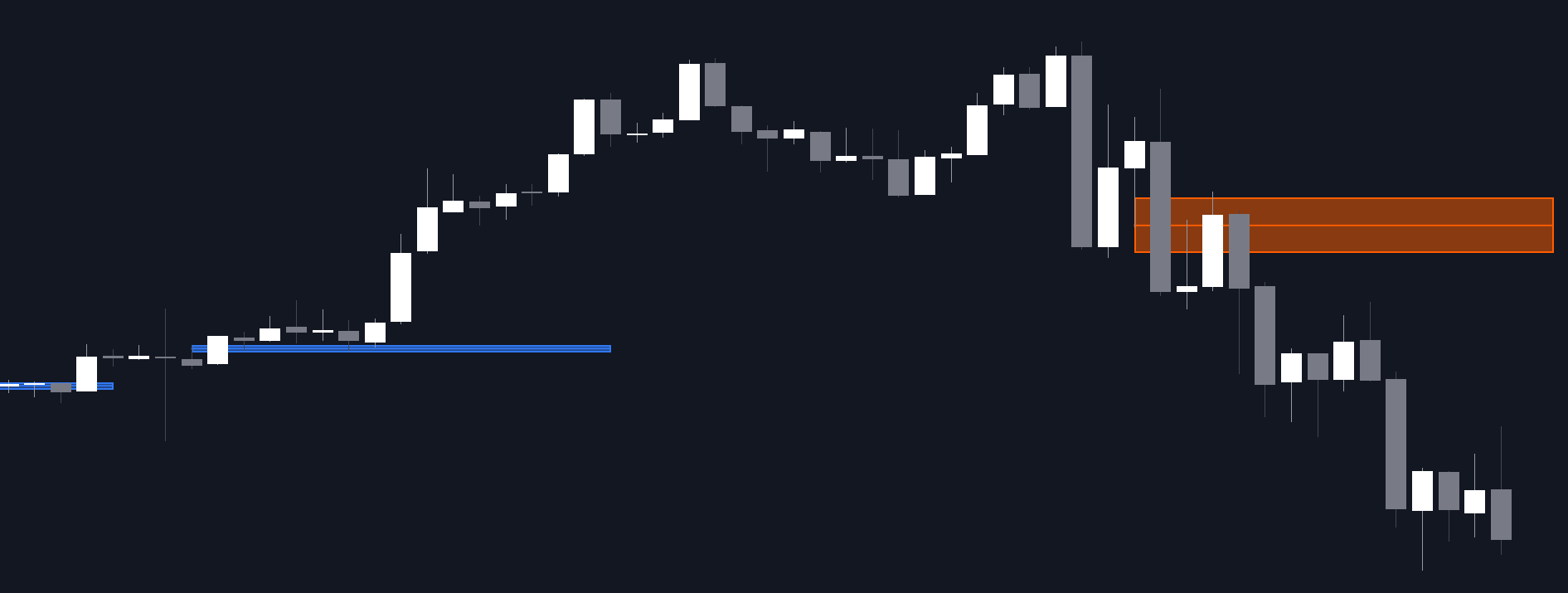# Moving Averages Proximity Oscillator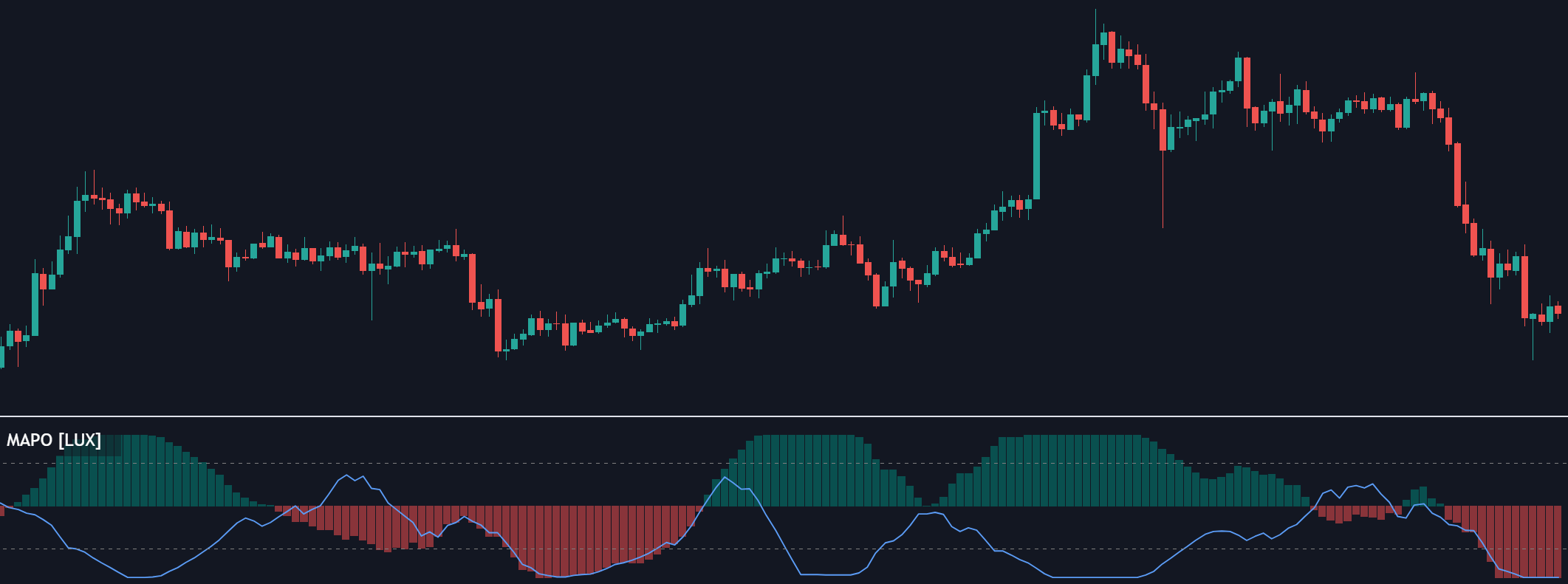This indicator returns the percentage or count of prices greater than simple moving averages with periods in a user set range, as well as the moving average period that is the closest to price values.

This indicator returns the percentage or count of prices greater than simple moving averages with periods in a user set range, as well as the moving average period that is the closest to price values.

#### Settings

• Minimum Length: Minimum SMA period
• Maximum Length: Maximum SMA period
• Smooth: Control the degree of smoothness of the indicator outputs
• Normalized: Normalize the indicator outputs in a range (0, 100)
• Src: Input source of the indicator

#### Usage

The indicator returns two outputs.

The "Price Above MA's" output returns for a current price value the number of times this one is greater than simple moving averages with periods ranging from Minimum Length to Maximum Length. This oscillator can be expressed as a percentage if Normalized is selected.

This oscillator allows identifying the direction of an underlying trend in the price. Higher Minimum Length and Maximum Length settings will return indications regarding longer term price variations, while shorter ranges will return less detailed outputs. This can be seen in the chart above where Minimum Length = 80 to Maximum Length = 100.

The "Proximity Index" output on the other end does not return information regarding the direction of an underlying trend but the period of the simple moving average with periods ranging from Minimum Length to Maximum Length that is the closest to the current price value.

For various simple moving averages of differing periods, we can see that SMA's with shorter periods will tend to stay closer to the price, when price start reverting it will reach higher periods moving averages.

As such, this second indicator output can help identify the start of new trends, with higher values indicating price is reverting toward longer-term moving averages, suggesting a new trend forming.

## Recent posts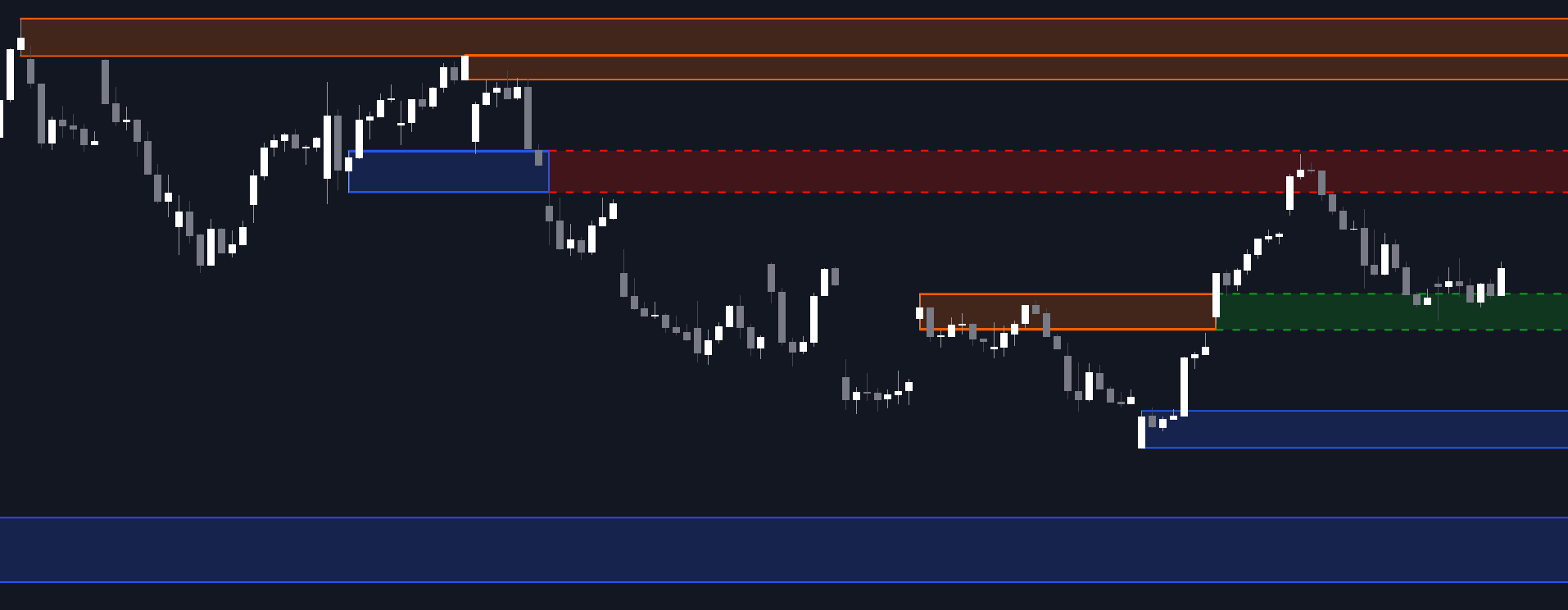### Order Blocks & Breaker Blocks

##### March 9, 2023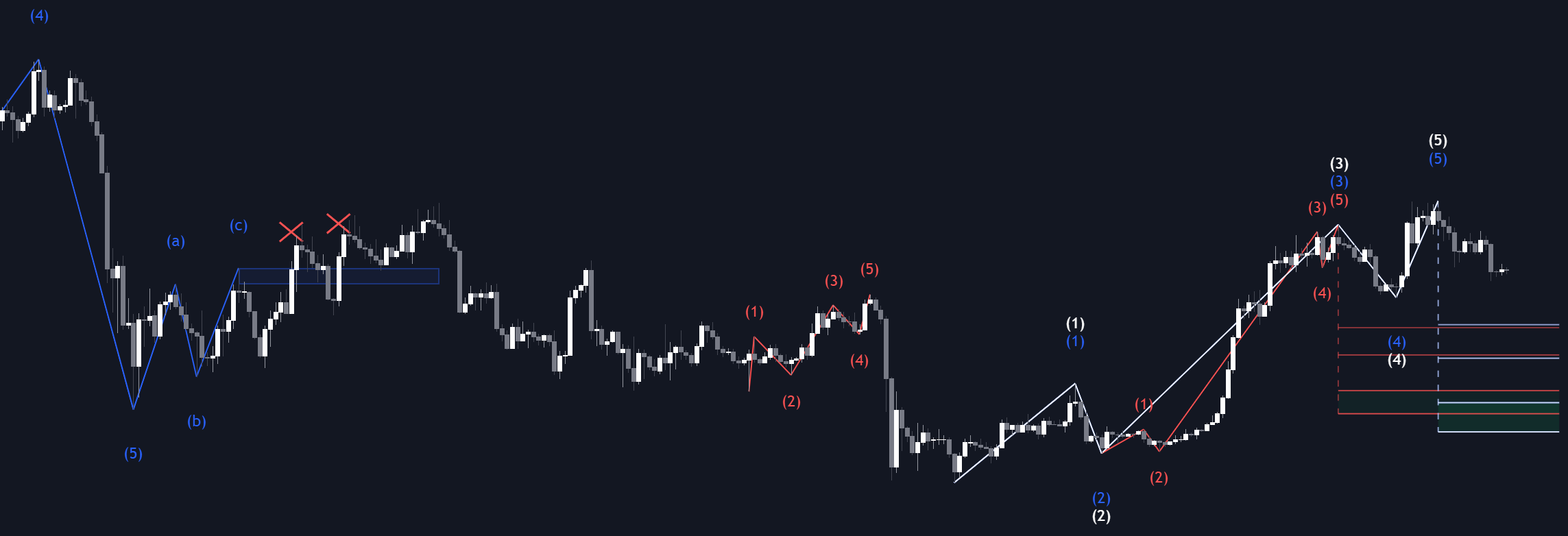### Elliott Wave

##### March 3, 2023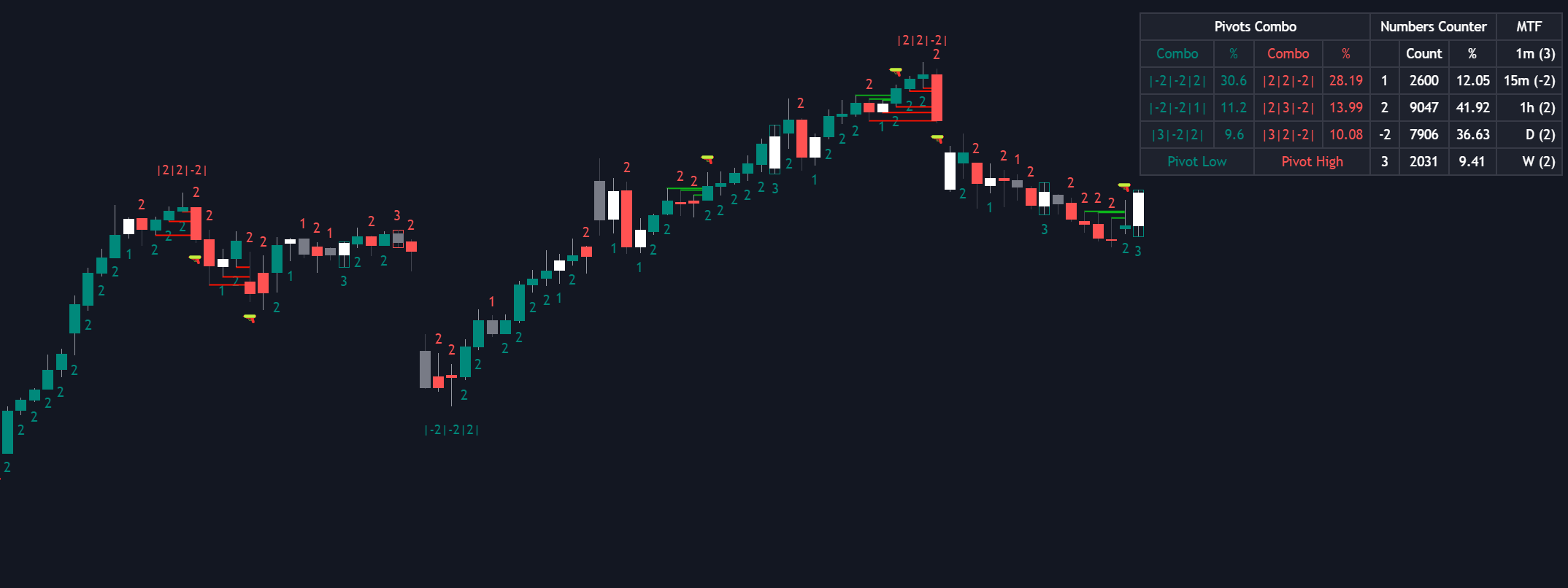### The Strat

##### March 2, 2023